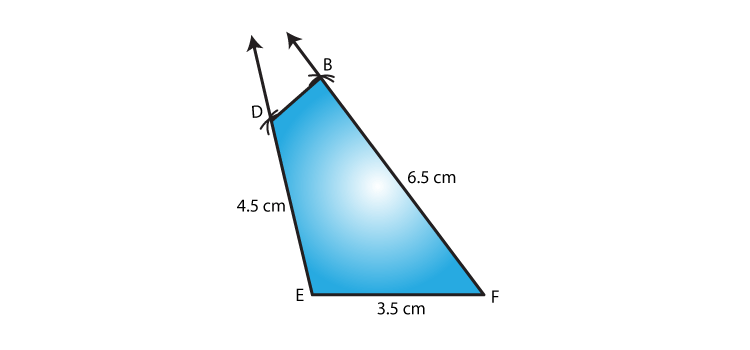# RD Sharma Solutions for Class 8 Maths Chapter 18 Practical Geometry (Constructions) Exercise 18.4

In Exercise 18.4, our expert faculty team have explained with examples the concept of construction of a quadrilateral when its three sides and their included angles are given. As the chapter is about constructions, students are advised to follow the steps to construct a quadrilateral which are explained by our experts in an easy manner. For better understanding, RD Sharma Class 8 Solutions pdf can be downloaded easily from the links given below.

## Download the Pdf of RD Sharma Solutions for Class 8 Maths Exercise 18.4 Chapter 18 Practical Geometry (Constructions)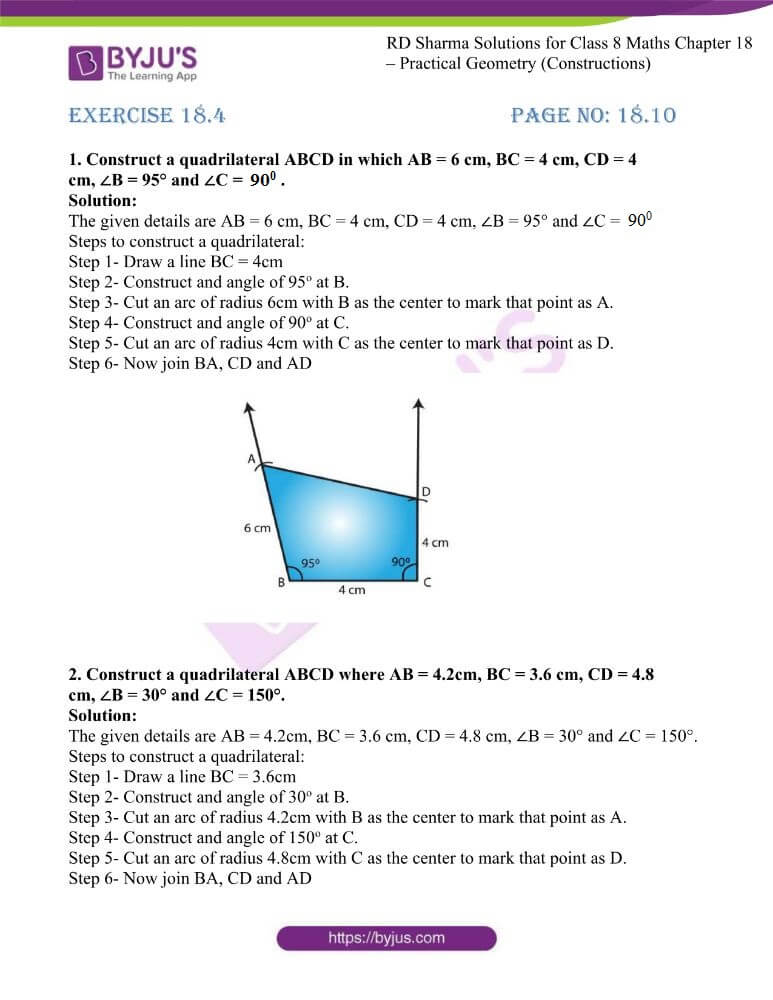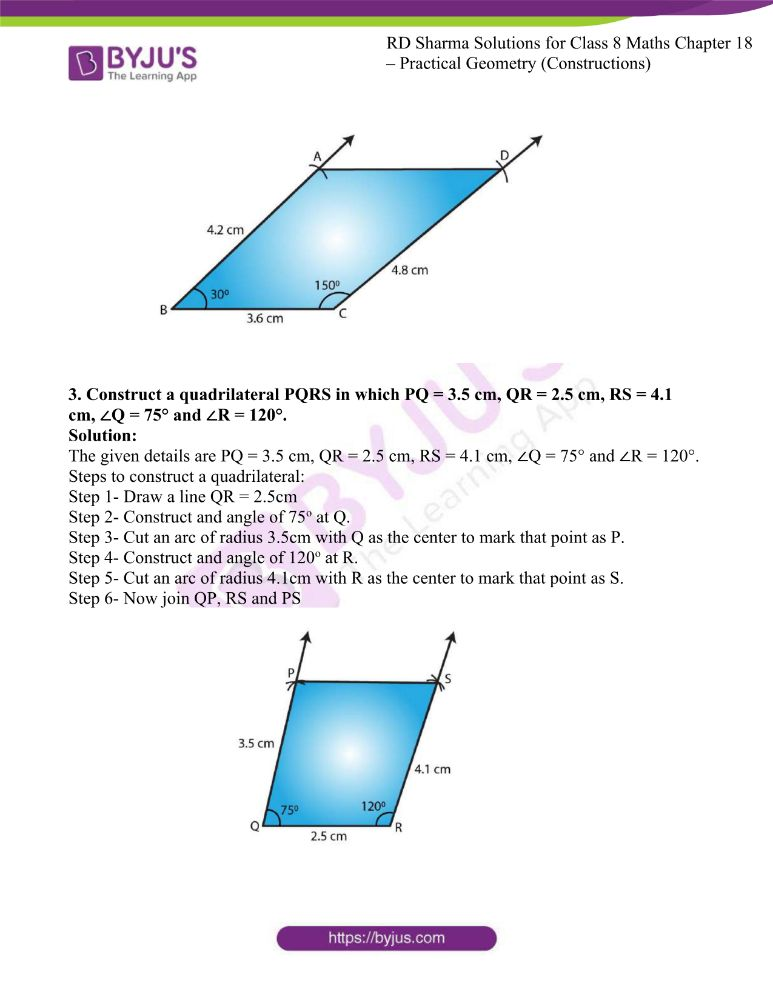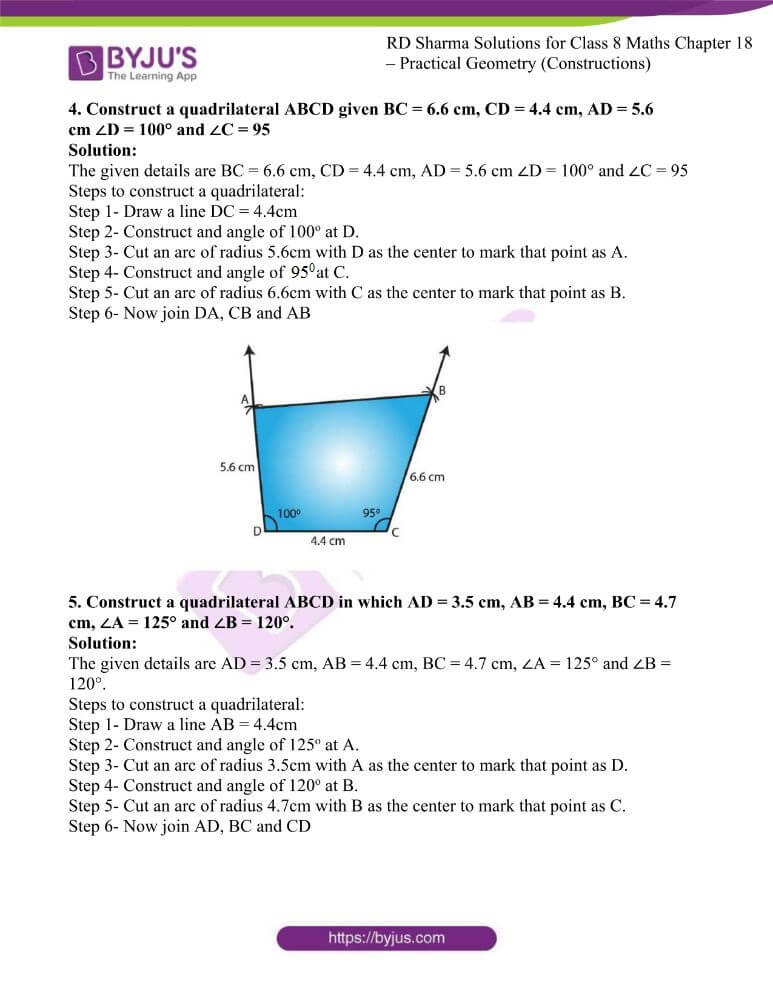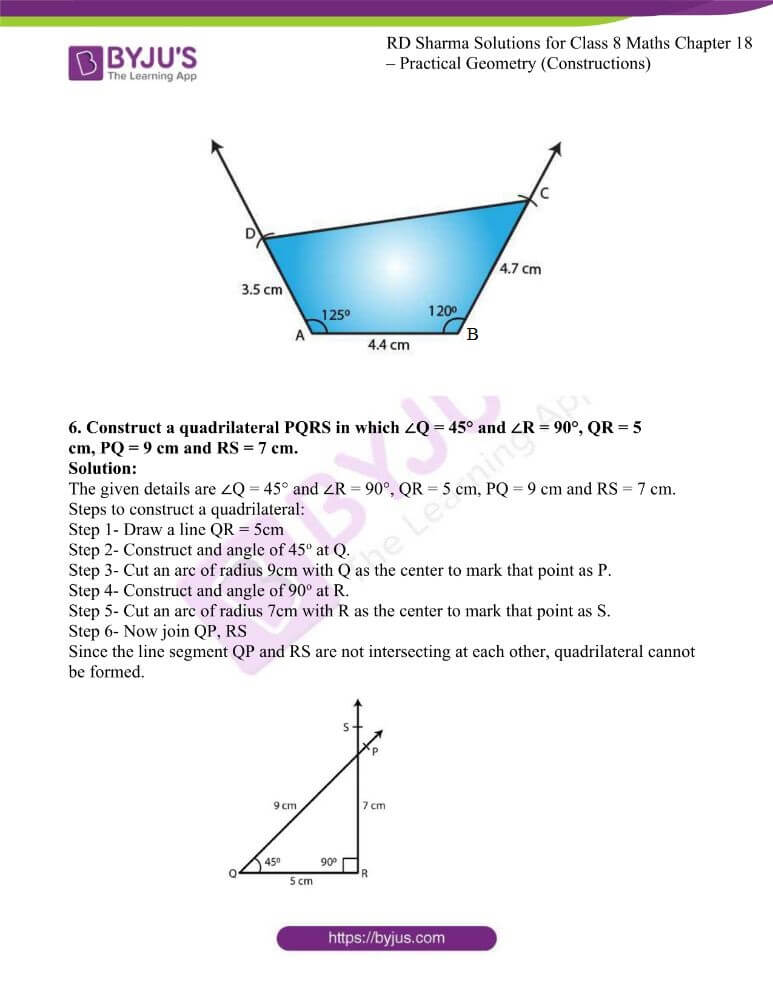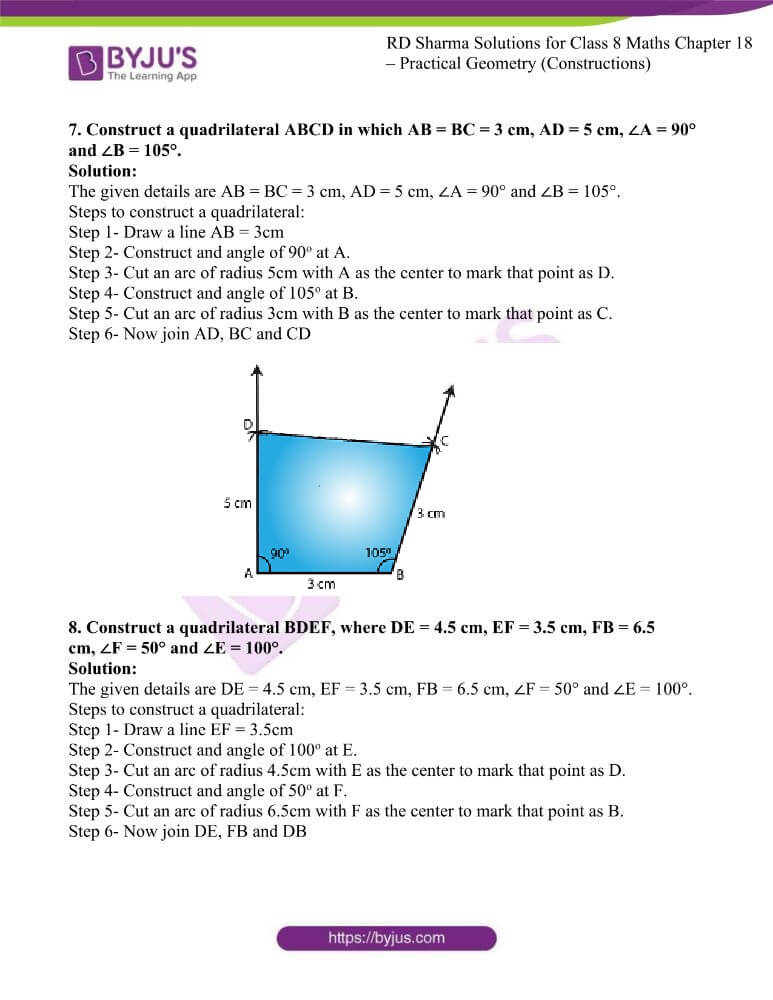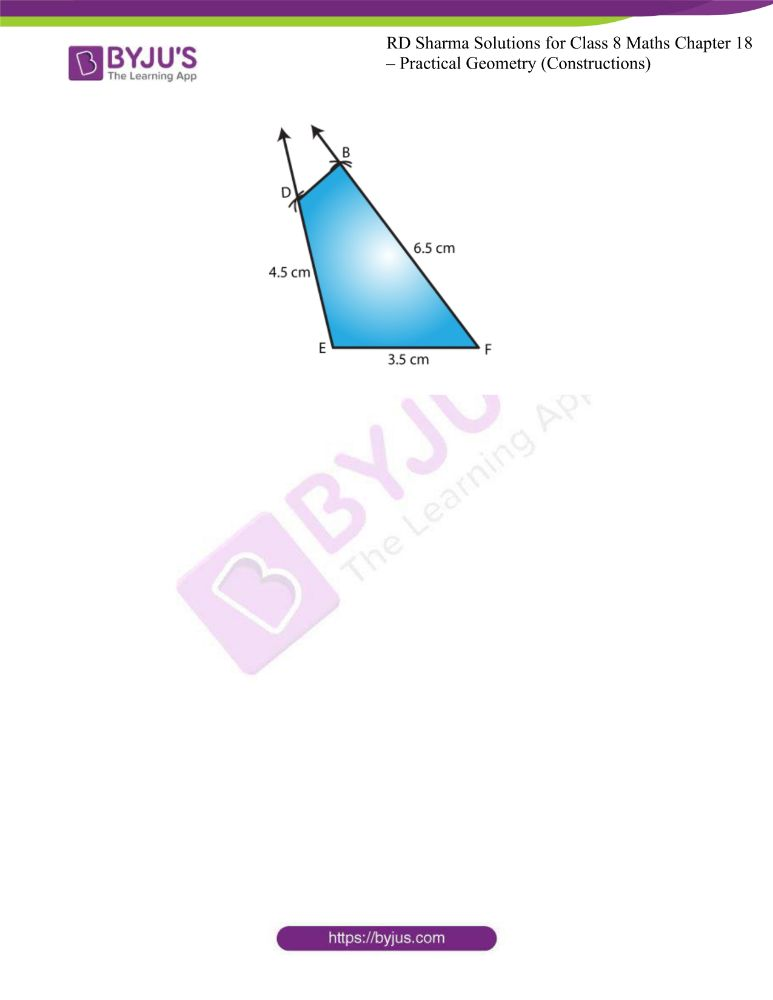### Access answers to RD Sharma Maths Solutions For Class 8 Exercise 18.4 Chapter 18 Practical Geometry (Constructions)

1. Construct a quadrilateral ABCD in which AB = 6 cm, BC = 4 cm, CD = 4 cm, ∠B = 95° and ∠C = 90°.

Solution:

The given details are AB = 6 cm, BC = 4 cm, CD = 4 cm, ∠B = 95° and ∠C = 90°.

Step 1- Draw a line BC = 4cm

Step 2- Construct and angle of 95o at B.

Step 3- Cut an arc of radius 6cm with B as the center to mark that point as A.

Step 4- Construct and angle of 90o at C.

Step 5- Cut an arc of radius 4cm with C as the center to mark that point as D.

Step 6- Now join BA, CD and AD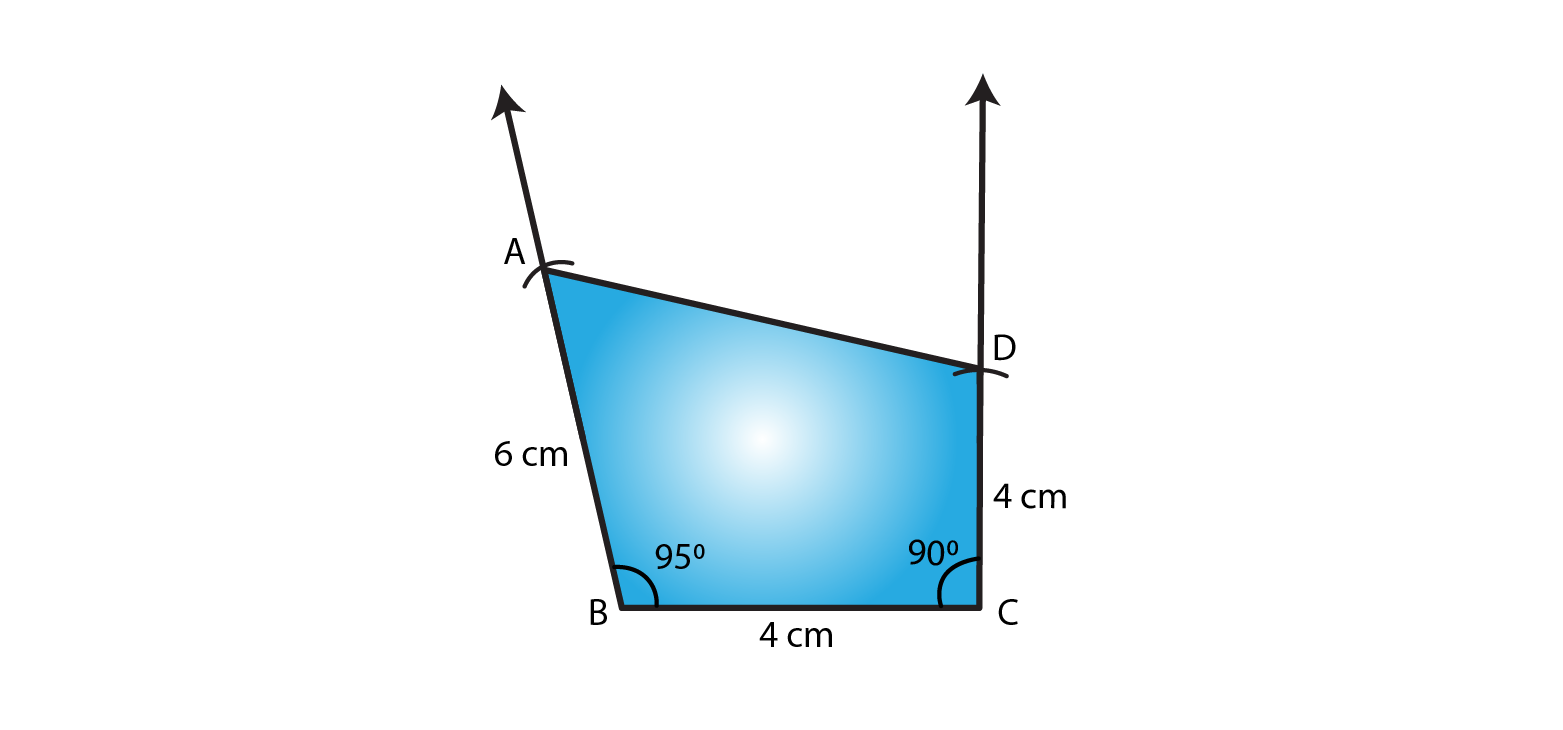2. Construct a quadrilateral ABCD where AB = 4.2cm, BC = 3.6 cm, CD = 4.8 cm, ∠B = 30° and ∠C = 150°.

Solution:

The given details are AB = 4.2cm, BC = 3.6 cm, CD = 4.8 cm, ∠B = 30° and ∠C = 150°.

Step 1- Draw a line BC = 3.6cm

Step 2- Construct and angle of 30o at B.

Step 3- Cut an arc of radius 4.2cm with B as the center to mark that point as A.

Step 4- Construct and angle of 150o at C.

Step 5- Cut an arc of radius 4.8cm with C as the center to mark that point as D.

Step 6- Now join BA, CD and AD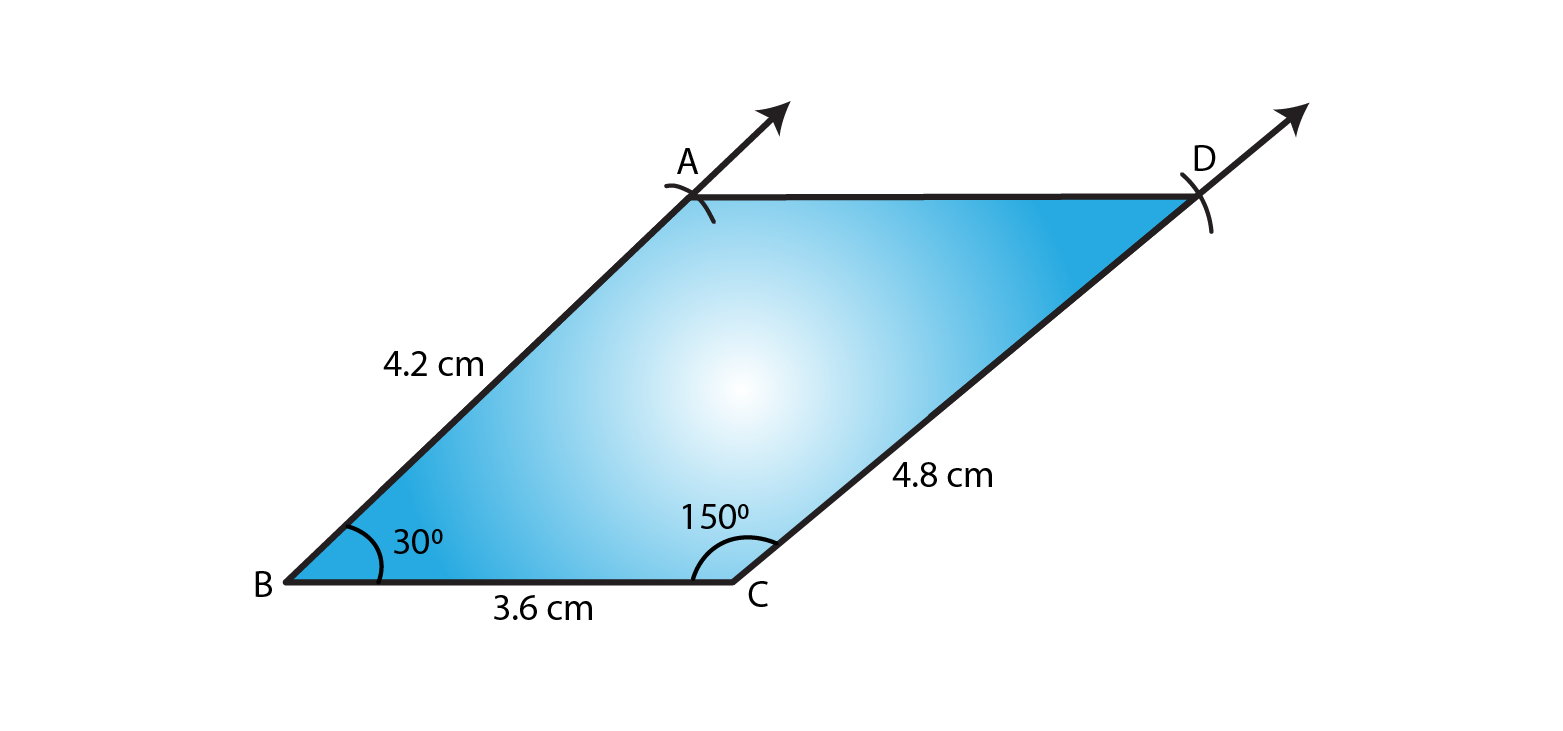3. Construct a quadrilateral PQRS in which PQ = 3.5 cm, QR = 2.5 cm, RS = 4.1 cm, ∠Q = 75° and ∠R = 120°.

Solution:

The given details are PQ = 3.5 cm, QR = 2.5 cm, RS = 4.1 cm, ∠Q = 75° and ∠R = 120°.

Step 1- Draw a line QR = 2.5cm

Step 2- Construct and angle of 75o at Q.

Step 3- Cut an arc of radius 3.5cm with Q as the center to mark that point as P.

Step 4- Construct and angle of 120o at R.

Step 5- Cut an arc of radius 4.1cm with R as the center to mark that point as S.

Step 6- Now join QP, RS and PS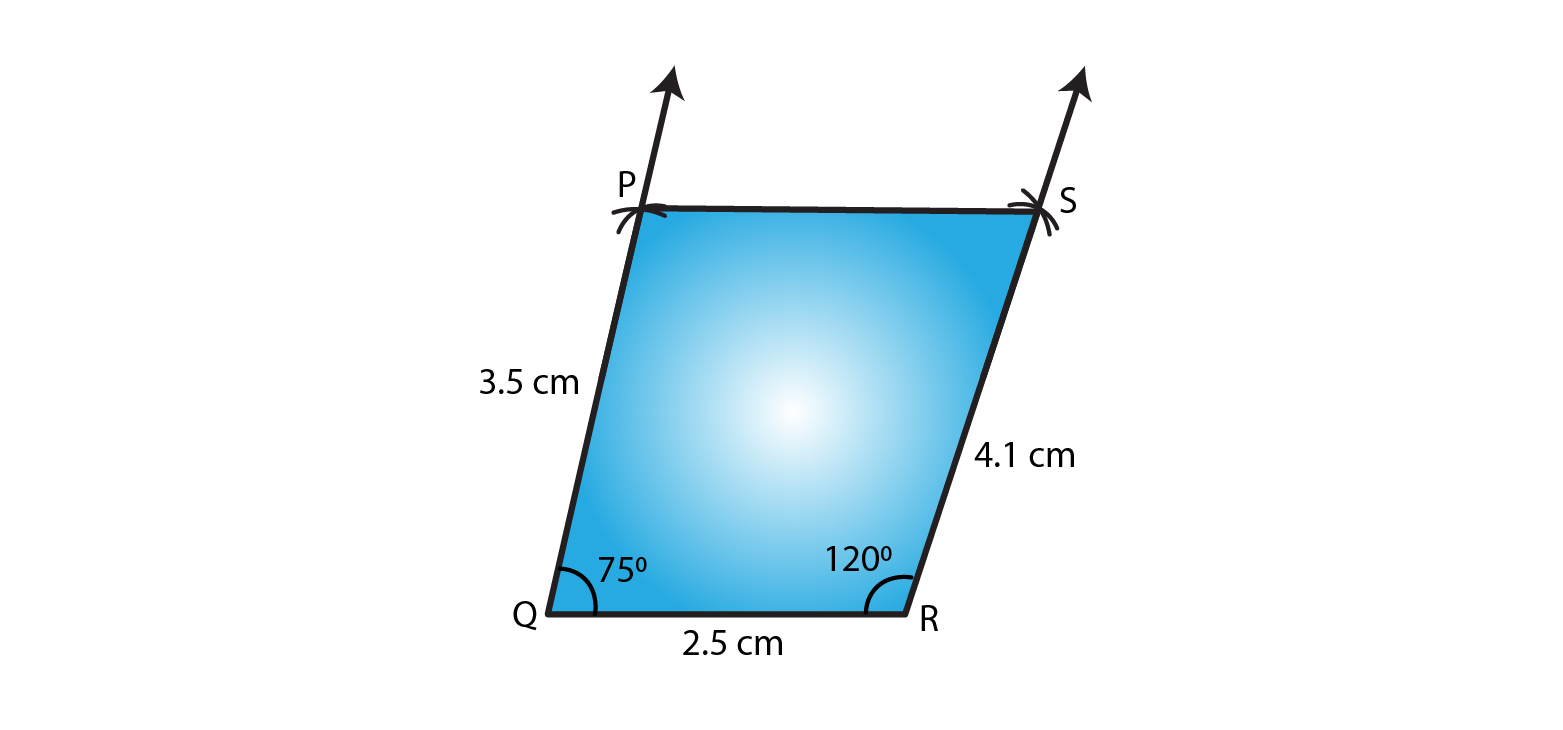4. Construct a quadrilateral ABCD given BC = 6.6 cm, CD = 4.4 cm, AD = 5.6 cm ∠D = 100° and ∠C = 95

Solution:

The given details are BC = 6.6 cm, CD = 4.4 cm, AD = 5.6 cm ∠D = 100° and ∠C = 95

Step 1- Draw a line DC = 4.4cm

Step 2- Construct and angle of 100o at D.

Step 3- Cut an arc of radius 5.6cm with D as the center to mark that point as A.

Step 4- Construct and angle of 95o at C.

Step 5- Cut an arc of radius 6.6cm with C as the center to mark that point as B.

Step 6- Now join DA, CB and AB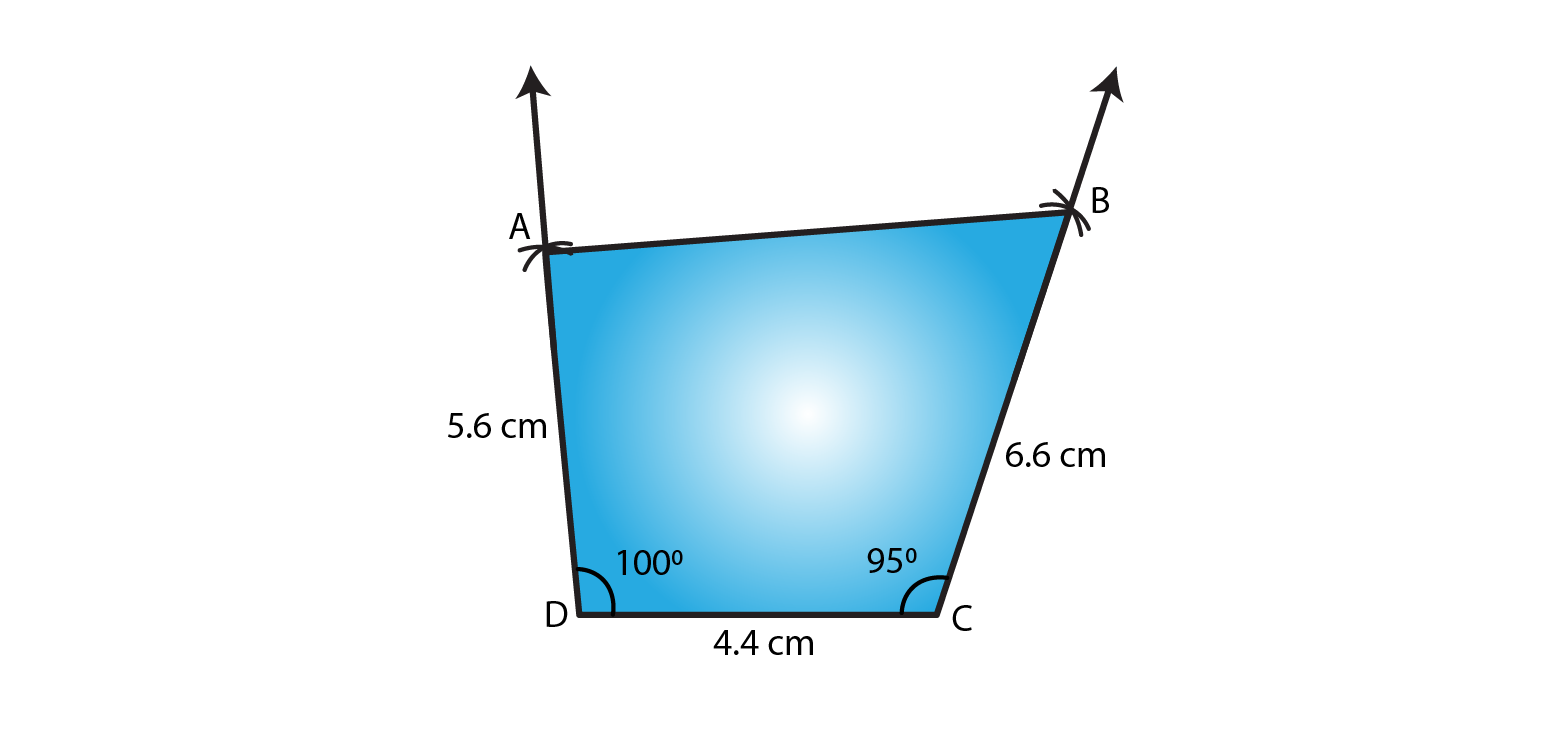5. Construct a quadrilateral ABCD in which AD = 3.5 cm, AB = 4.4 cm, BC = 4.7 cm, ∠A = 125° and ∠B = 120°.

Solution:

The given details are AD = 3.5 cm, AB = 4.4 cm, BC = 4.7 cm, ∠A = 125° and ∠B = 120°.

Step 1- Draw a line AB = 4.4cm

Step 2- Construct and angle of 125o at A.

Step 3- Cut an arc of radius 3.5cm with A as the center to mark that point as D.

Step 4- Construct and angle of 120o at B.

Step 5- Cut an arc of radius 4.7cm with B as the center to mark that point as C.

Step 6- Now join AD, BC and CD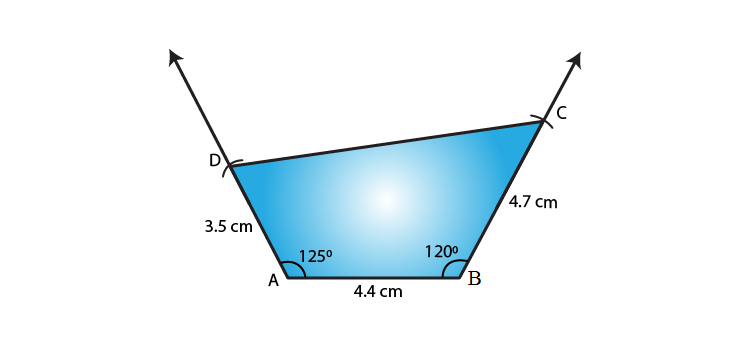6. Construct a quadrilateral PQRS in which ∠Q = 45° and ∠R = 90°, QR = 5 cm, PQ = 9 cm and RS = 7 cm.

Solution:

The given details are ∠Q = 45° and ∠R = 90°, QR = 5 cm, PQ = 9 cm and RS = 7 cm.

Step 1- Draw a line QR = 5cm

Step 2- Construct and angle of 45o at Q.

Step 3- Cut an arc of radius 9cm with Q as the center to mark that point as P.

Step 4- Construct and angle of 90o at R.

Step 5- Cut an arc of radius 7cm with R as the center to mark that point as S.

Step 6- Now join QP, RS

Since the line segment QP and RS are not intersecting at each other, quadrilateral cannot be formed.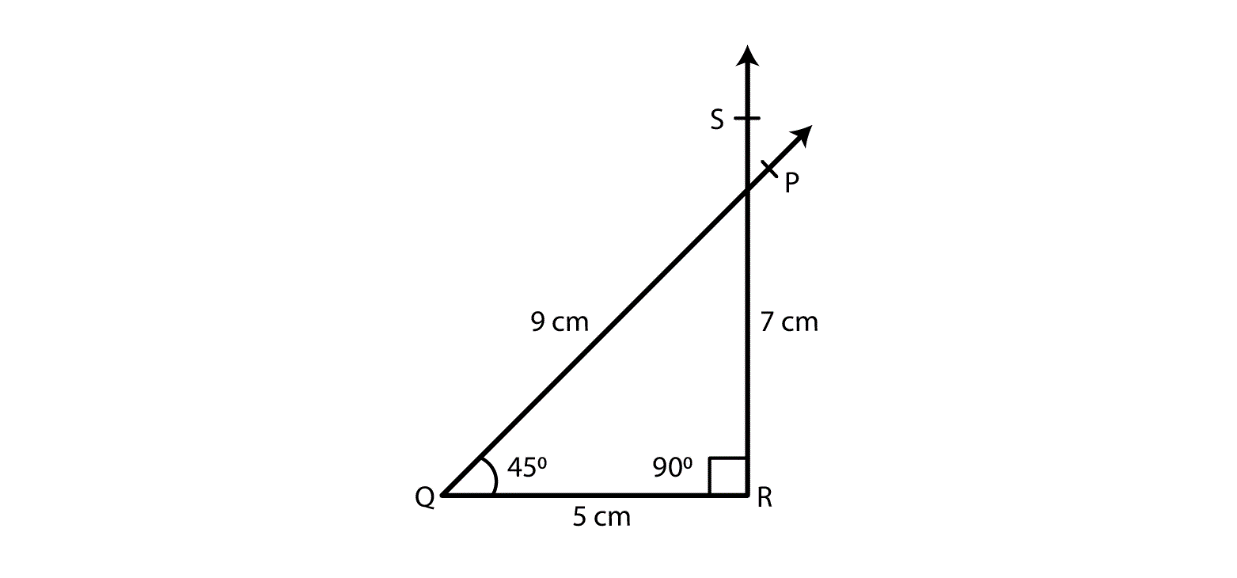7. Construct a quadrilateral ABCD in which AB = BC = 3 cm, AD = 5 cm, ∠A = 90° and ∠B = 105°.

Solution:

The given details are AB = BC = 3 cm, AD = 5 cm, ∠A = 90° and ∠B = 105°.

Step 1- Draw a line AB = 3cm

Step 2- Construct and angle of 90o at A.

Step 3- Cut an arc of radius 5cm with A as the center to mark that point as D.

Step 4- Construct and angle of 105o at B.

Step 5- Cut an arc of radius 3cm with B as the center to mark that point as C.

Step 6- Now join AD, BC and CD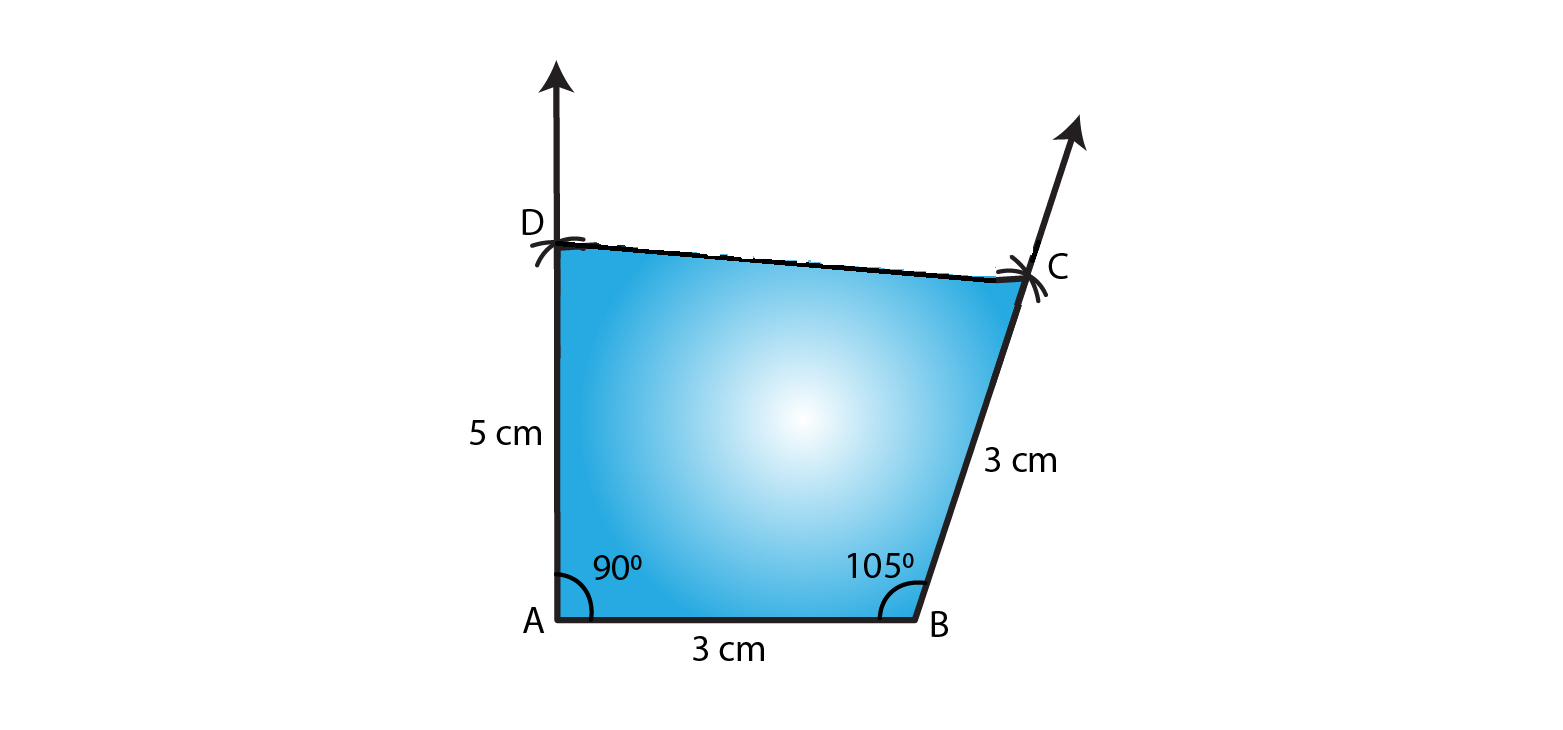8. Construct a quadrilateral BDEF, where DE = 4.5 cm, EF = 3.5 cm, FB = 6.5 cm, ∠F = 50° and ∠E = 100°.

Solution:

The given details are DE = 4.5 cm, EF = 3.5 cm, FB = 6.5 cm, ∠F = 50° and ∠E = 100°.

Step 1- Draw a line EF = 3.5cm

Step 2- Construct and angle of 100o at E.

Step 3- Cut an arc of radius 4.5cm with E as the center to mark that point as D.

Step 4- Construct and angle of 50o at F.

Step 5- Cut an arc of radius 6.5cm with F as the center to mark that point as B.

Step 6- Now join DE, FB and DB